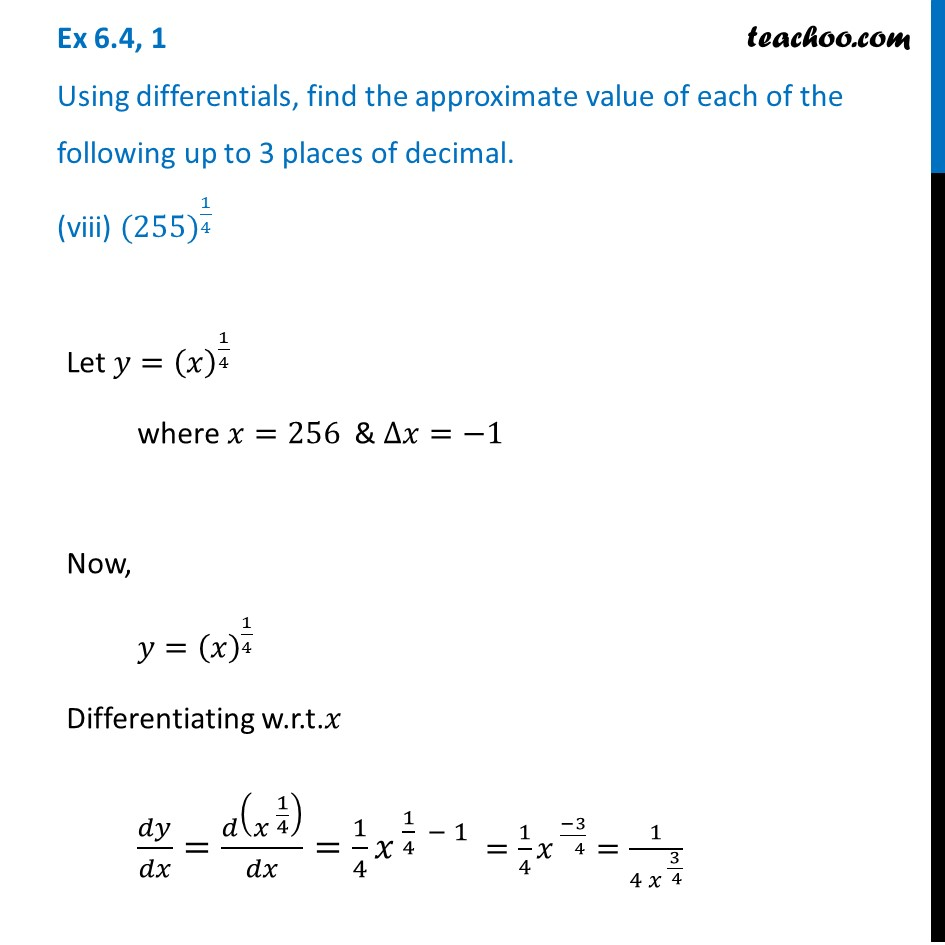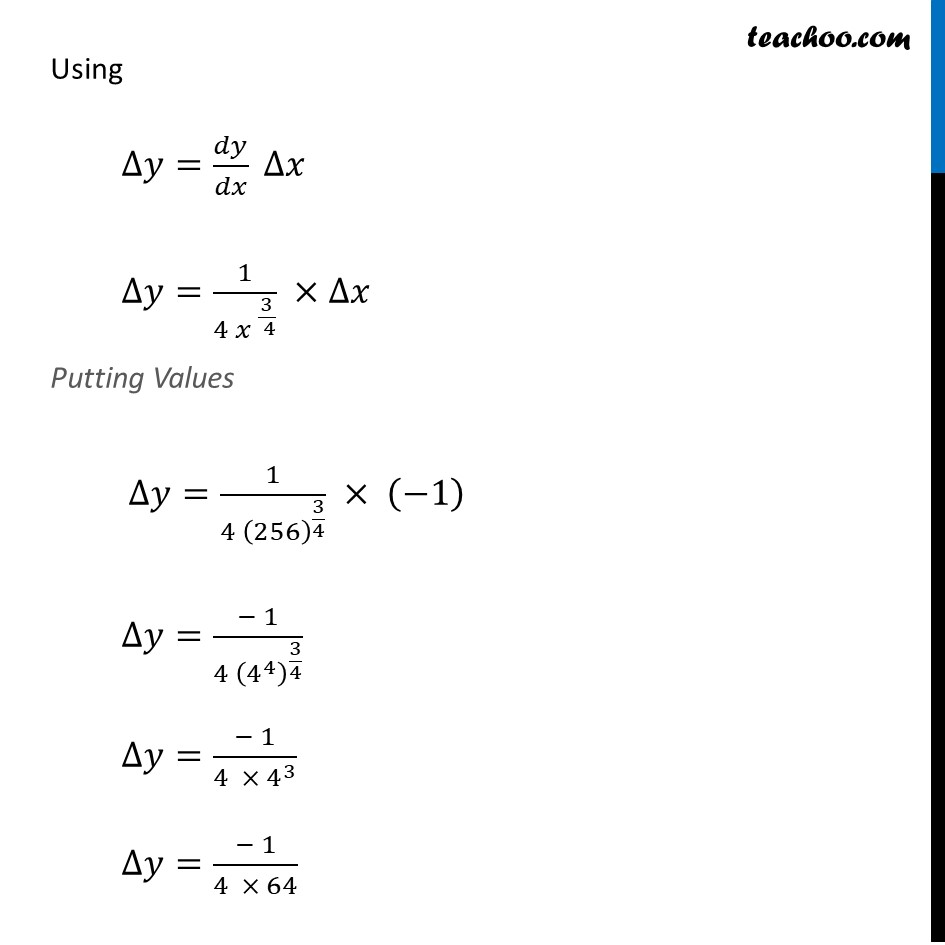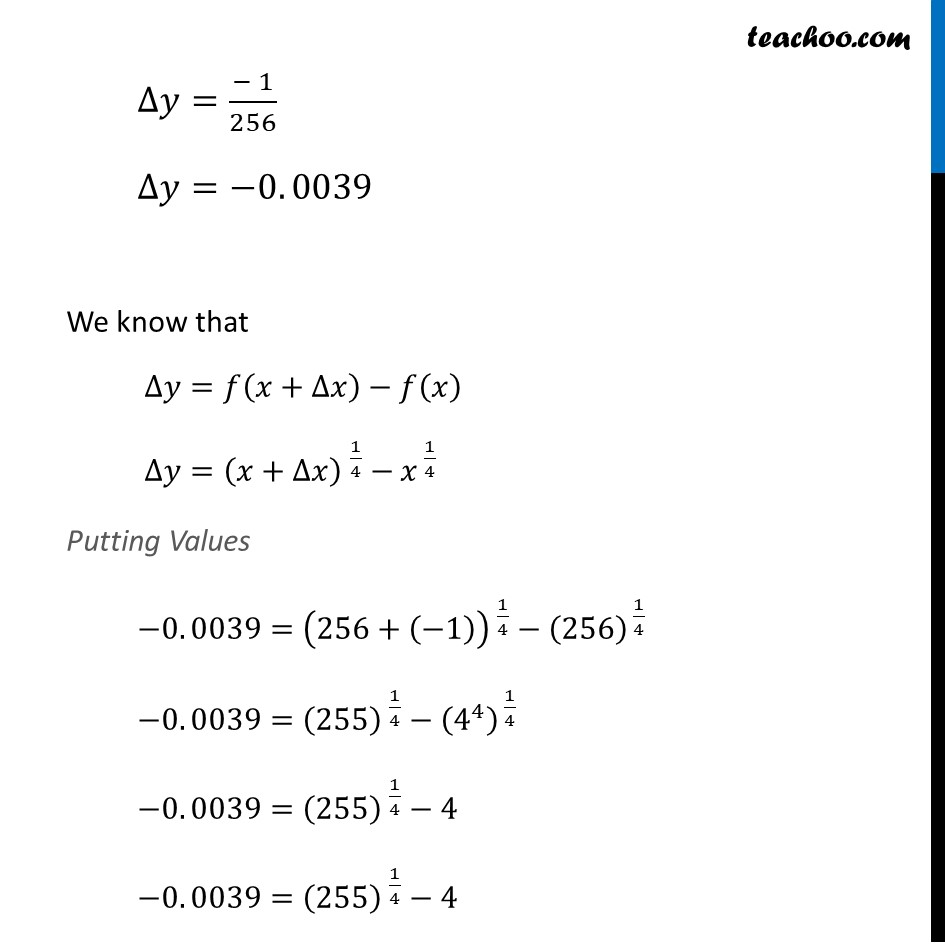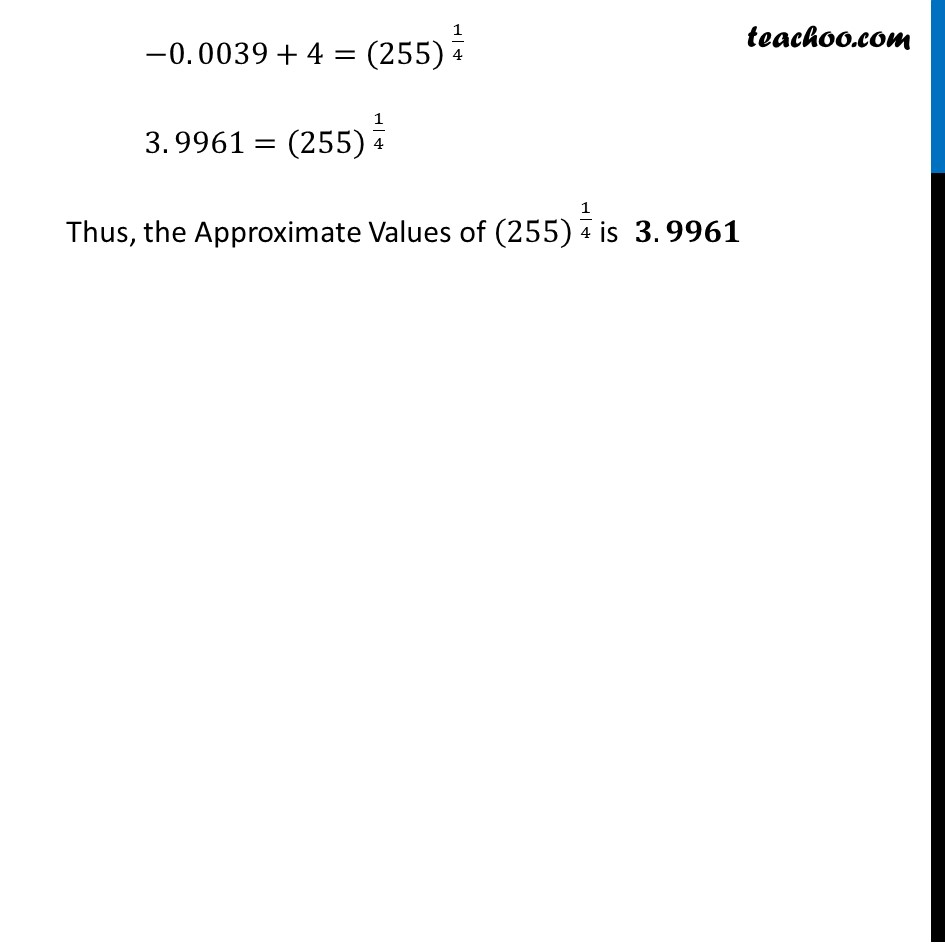Approximations (using Differentiation)

Chapter 6 Class 12 Application of Derivatives
Serial order wiseLearn in your speed, with individual attention - Teachoo Maths 1-on-1 Class

### Transcript

Question 1 Using differentials, find the approximate value of each of the following up to 3 places of decimal. (viii) 〖(255)〗^(1/4)Let 𝑦=(𝑥)^(1/4) where 𝑥=256 & ∆𝑥=−1 Now, 𝑦=(𝑥)^(1/4) Differentiating w.r.t.𝑥 𝑑𝑦/𝑑𝑥=𝑑(〖𝑥 〗^(1/4) )/𝑑𝑥=1/4 𝑥^( 1/4 − 1) =1/4 𝑥^( (−3)/( 4))=1/(4 𝑥^( 3/( 4)) ) Using ∆𝑦=𝑑𝑦/𝑑𝑥 ∆𝑥 ∆𝑦=1/(4〖 𝑥〗^( 3/( 4)) ) ×∆𝑥 Putting Values ∆𝑦=1/(4 (256)^(3/4) ) × (−1) ∆𝑦=(− 1)/(4 (4^4 )^(3/4) ) ∆𝑦=(− 1)/(4 × 4^3 ) ∆𝑦=(− 1)/(4 × 64) ∆𝑦=(− 1)/256 ∆𝑦=−0. 0039 We know that ∆𝑦=𝑓(𝑥+∆𝑥)−𝑓(𝑥) ∆𝑦=〖(𝑥+∆𝑥) 〗^(1/4)−𝑥^( 1/4) Putting Values −0. 0039=〖(256+(−1)) 〗^(1/4)−(256)^( 1/4) −0. 0039=〖(255) 〗^(1/4)−(4^4 )^( 1/4) −0. 0039=〖(255) 〗^(1/4)−4 −0. 0039=〖(255) 〗^(1/4)−4 −0. 0039+4=〖(255) 〗^(1/4) 3. 9961=〖(255) 〗^(1/4) Thus, the Approximate Values of 〖(255) 〗^(1/4) is 𝟑. 𝟗𝟗𝟔𝟏